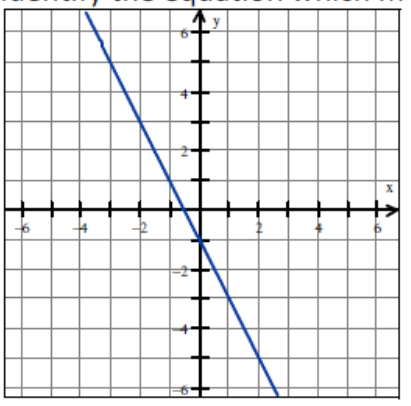Mathematics
Easy

Question

# Identify the equation which matches the following graph## Y = 2x – 1X2 + y2 = 1Y = –2x – 1Y = x2 - 1Hint:

## The correct answer is: Y = –2x – 1

### GIVEN-The given line passes through the points (-1,1) & (0,-1) as shown in the given graph.TO FIND-Equation of the given line.SOLUTION-From the given graph, we observe that-(-1,1) & (0,-1) are 2 points on the line.We know that when a line passes through a given point, the coordinates of that point satisfies the equation of the said line.Hence, we substitute the coordiantes of both the points in the given options to find the correct equation, for which LHS = RHS.a. y = 2x - 1We substitute (-1,1) in the given equation-   y = 2x - 1∴ 1 = 2 (-1) - 1∴ 1 = -2 - 1∴ 1 ≠ -3∴ LHS ≠ RHSSince (-1,1) does not satisfy the given equation, y = 2x - 1 is not the equation of the given line.b. x2 + y2 = 1We substitute (-1,1) in the given equation-         x2 + y2 = 1∴ (-1)2 + (1)2 = 1∴          1 + 1 = 1∴                2 ≠ 1∴           LHS ≠ RHSSince, (-1,1) does not satisfy the given equation, x2 + y2 = 1 is not the equation of the given line.c. y = -2x - 1We substitute (-1,1) in the given equation-   y = -2x - 1∴ 1 = -2 (-1) - 1∴ 1 = 2 - 1∴ 1 = 1∴ LHS = RHSWe substitute (0,-1) in the given equation-    y = -2x - 1∴ -1 = -2 (0) - 1∴ -1 = 0 - 1∴ -1 = -1∴ LHS = RHSSince both (-1,1) and (0,-1) satisfy the given equation, y = -2 x - 1 is the equation of the given line.FINAl ANSWER-Option 'c' i.e. 'y = -2x - 1' is the correct answer to the given question.

Alternatively, we can use the 2 point formula to find the equation of the given line-
When we have 2 points that lie on a given line then we can find the equation of the said line by using the 2-point formula-
(y-y1) = (y2-y1) * (x-x1)
(x2-x1)
In the given question, x1 = -1, y1 = 1, x2 = 0 & y2 = -1
∴ (y-y1) = (y2-y1) * (x-x1)
(x2-x1)
∴ (y - 1) = (-1 - 1) * [x - (-1)]
[0 - (-1)]
∴ (y - 1) =  -2      * (x + 1)
0 + 1
∴ (y - 1) = -2/1 * (x + 1)
∴ y - 1 = -2x - 2
∴ y = -2x - 2 + 1
∴ y = -2x - 1

### Related Questions to study#### With Turito Foundation.#### Get an Expert Advice From Turito.